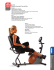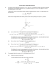# 1 Two protons move parallel to x- axis in opposite

## Transcription

1 Two protons move parallel to x- axis in opposite
```Slide 1 / 30
1
Two protons move parallel to x- axis in opposite
directions at the same speed v. What is the
direction of the magnetic force on the upper
proton?
A to the right
B to the left
C out of the page
D into the page
E to the top of the page
Slide 2 / 30
2
A positive charge q moves at a constant speed v
in the direction presented by the above diagram.
What is the direction of the magnetic field due to
the moving charge at point P?
A to the right
B to the left
C out of the page
D into the page
E to the top of the page
Slide 3 / 30
3
A negative charge q moves at a constant speed v
in the direction presented by the above diagram.
What is the direction of the magnetic field due to
the moving charge at point P?
A to the right
B to the left
C out of the page
D into the page
E to the bottom of the page
Slide 4 / 30
4
A positive charge q moves at a constant speed v
parallel to the x-axis. At which of the following
points is the magnetic field strongest in
magnitude?
A A
B B
C C
D D
E E
Slide 5 / 30
5
Two positive charges of equal magnitude move in
a reference frame shown on the above diagram.
What is the direction of the net magnetic field at
the origin?
A into the page
B out of the page
C to the left
D to the right
E zero
Slide 6 / 30
6
Two opposite charges of equal magnitude move
in a reference frame shown on the diagram. What
is the direction of the net magnetic field at the
origin?
A into the page
B out of the page
C to the left
D to the right
E zero
Slide 7 / 30
7
A long, straight wire, shown above, carries an
electric current I. What is the direction of the
magnetic field at point P?
A into the page
B out of the page
C to the right
D to the left
E to the top of the page
Slide 8 / 30
8
A long, straight wire carries an electric current I
directed into the page. If B0 is the magnitude of
magnetic field due the current at point A, what is
the magnitude of the field at point B?
A
B
C
D
E
Slide 9 / 30
9
Two long, straight wires carry equal currents
directed into the page. What is the direction of the
magnetic field at the midpoint between the wires?
A into the page
B out of the page
C to the top of the page
D to the bottom of the page
E zero
Slide 10 / 30
10
Two long, straight wires carry equal currents
directed into the page. The wires are placed at
two corners of an equilateral triangle. Which of
the following is the correct direction of the net
magnetic field at point P?
A
B
C
D
E
Slide 11 / 30
11
Two long, straight wires carry equal, but opposite,
currents perpendicular to the page as shown
above. The wires are placed at two corners of an
equilateral triangle. Which of the following is the
correct direction of the net magnetic field at point
P?
A
B
C
D
E
Slide 12 / 30
Slide 13 / 30
Slide 14 / 30
14
Two long, straight wires carry equal currents
perpendicular to the page. There is an attractive
magnetic force between the wires of magnitude
F0. What is the new force between the wires if the
magnitude of each current is doubled?
A
B
C
D
E
Slide 15 / 30
15
Two long, straight wires carry equal currents
perpendicular to the page. There is an attractive
magnetic force between the wires of magnitude
F0. What is the new force between the wires if the
distance between the wires is doubled?
A
B
C
D
E
Slide 16 / 30
16
Three parallel wires are each carrying a current I
in the direction shown on the diagram. What is the
direction of the net magnetic force on the bottom
wire?
A out of the page
B into the page
C to the top of the page
D to the bottom of the page
E zero
Slide 17 / 30
17
Two circular loops of wire each carry a current I.
Loop A has twice the radius of loop B. What is the
ratio of the magnetic field at the center of loop A
to that of loop B?
A
B
C
D
E
Slide 18 / 30
18
A circular conductor carries an electric current I.
The loop is placed in the x-y-z reference frame in
such way that the center of the loop is at the
origin. The plane of the loop is parallel to the y-z
axes and perpendicular to the x-axis. Which one
of the following graphs represents the magnetic
field strength along the x-axis?
A
C
B
D
E
Slide 19 / 30
Slide 20 / 30
Slide 21 / 30
Slide 22 / 30
Slide 23 / 30
23
A uniform magnetic field B is created by the
electric current flowing through a long solenoid.
What is the magnitude of the magnetic field inside
the solenoid with the same current and twice the
A
B
C
D
E
Slide 24 / 30
Slide 25 / 30
Slide 26 / 30
26
A uniform magnetic field B is created by an
electric current I flowing through a long solenoid
with n turns per unit length and radius R. What is
the magnitude of the magnetic field inside the
solenoid?
A
B
C
D
E
Slide 27 / 30
27
A cylindrical conductor with radius a carries an
electric current I. The current is uniformly
distributed over the cross-sectional area of the
cylinder. Which of the following graphs
represents the magnetic field B as a function of
distance r, both inside and outside the conductor?
A
C
B
D
E
Slide 28 / 30
28
A solid conductor with radius a is surrounded by
a tube with an inner radius b and outer
c. The conductor and tube carry equal currents in
opposite directions (conductor - out of the page,
tube - into the page). An electron e travels to the
right in the space between the conductor and tube
at point P. What is the direction of the magnetic
force on the electron at point P?
A Into the page
B out of the page
C to the bottom of the page
D to the top of the page
E to the left
Slide 29 / 30
Slide 30 / 30
```

### Problem Set 08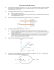### PHYSQ 126, Quiz 6 (19 mars 2015) Interaction of a Current Loop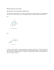### Ph 632 Problem Set #4 - Department of Physics | Oregon State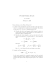### Medizinische Austellung A4 Wien 2008.indd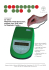### PHYS1250 Fundamental Physics Assignment Three Due Date: 5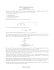### Are You Stuck on 3D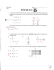### Physics 112 Sample Test 2 NAME __________________________